## Upcoming Guests on Inside the Eye - Live!

Visit Inside the Eye - Live!, the new website for Inside the Eye - Live! radio show with The Fetch!

Show starts at 10:00am Eastern Call in or listen live!

Show Archives (including show summaries) from Apr 1, 2013. Previous show archives (without show summaries here).

Important note to visitors. Blogger has changed the HTML code used when this blog was originally created. What that means is that some pictures may not load, or pictures have disappeared from original linking URL's and are no longer available. Fixing any picture requires an entire reload of all pictures so that the pictures work with HTTPS, or Blogger will not allow any in story photo updates. Pages are currently being updated (freshened up). Be patient as pages get updated. Updates are based on various page use/visit priorities.

## Friday, August 29, 2008

### English Gematria and the Order of the Universe

"Things" are not as they appear and truth as it has been crafted is not so difficult to penetrate nor so difficult to pierce - if only you had the knowledge and the education to see through the Construct. Let us blast through some of the basics.

1) We hold that mankind has been drowned. As such, we hold no difference, phonetically and hence esoterically, between the global mythology known as "the Deluge" and that which constitutes a state of "Delusion". The very design in the phonetics holds the core or key of the fabrication.

2) We hold that the "Word" is "Pi" and that English is a hyper-dimensional representation of this reality. As 1 is to the 0, so the male is to the female. Each cannot exist without the other.

3) Gematria is an Occult Science as well as an Occult Art. Gematria is the willful or conscious embedding of esoteric formulae into the language with the intent to hide and yet reveal the core of the Construct, while ART is used as a means of interpretation.

"For the mode of any more abstruse experiment, we explain it,
Lest any mistake arise about it; and to the intent, also,
That we may excite others to excogitate better methods
." - Sir Francis Bacon, Alphabet of Nature

Example:

ISIS = 9+19+9+19 = 56 = 19+16+5+5+3+8 = SPEECH
OSIRIS = 15+19+9+18+9+19 = 89 = 18+5+12+9+7+9+15+14 = RELIGION

In the Oral tradition, we twist the L

4) The Construct is wedded, or embedded, into Nature. In Nature is revealed all things.

"In Nature's infinite Book of secresy
A little I have read."--Antony & Cleopatra i.2"

5) Symbols as Letters are two dimensional representation of 3 or more dimensional concepts. Example: D is as the Sun as it appears on the HORIZON. The word HORIZON is a CODE for the following:

H = Greek Letter Pi Transposition
ORIZ = ZIRO = ZERO
ON = ON(e) = OneHORIZON as an ESOTERIC CONSTRUCT embeds the code of "Pi (as the Letter H) is comprised of the ZERO and the ONE. The Letter D, being a 90 degree turn of the Sun as it appears to rise on the HORIZON, thus becomes the 3+ dimensional representation of this conceptual.

Esoteric English and the Pi Proportion

Of all the axioms held there remains the primary axiom: Pi is the building block upon which the whole of the Illuminatus system is predicated. If you keep this core thought in mind as you delve further into your own research, this reality will become ever more profound and clear.

However, let us aid in the illumination:

One book you should equate yourself with is "The Beginning of Masonry" by Higgins. He writes in the chapter called "The Pi Proportion",

"It is completely in accord with the ancient philosophy, which, in apostrophizing the Deity as the "Pi proportion", meant what later, in Isaiah, is called "The living Elohim" - The Pi proportion, endowed with omnipotent self-exertion in the ordering of inchoate matter; in other words, a LOGOS active in Cosmos. The Pi proportion is something that is never absent, in one form or other, from every one of the world's primitive religions, and certainly enters deeply and radically into the philosophies that have given rise to what we in these days call "Masonry".

Elsewhere, this excerpt is noted: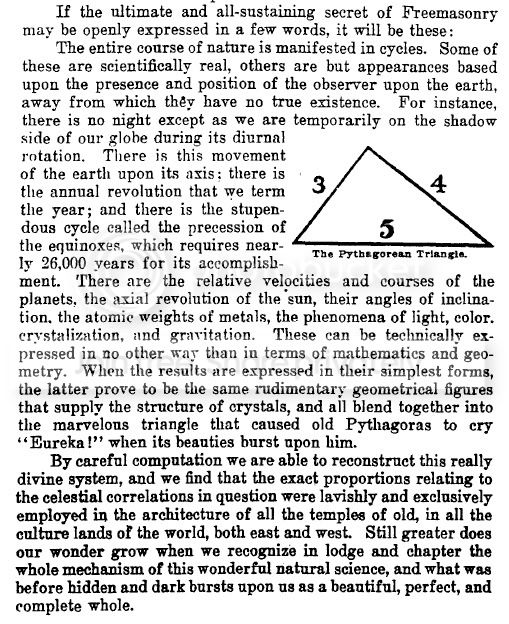The Pi Proportion, infused as it is "into all things", would of necessity, then, be infused into the very words which comprise the language that such Mason's were crafting, or so it would seem. Diurnal orbits, planetary speeds, speed of light which have clearly been embed into sacred architecture, would be embed into the English language itself.

To understand how this might be so, the concept known as the Pi proportion has to be understood. Fortunately, it is very, very, simple. The 1 as a diameter cannot exist without the circumference. 1's are encoded as male and 0's are encoded as female. Each cannot exist without the other. This "stupendous mechanism" is only stupendous when set against Jehovian, or Setian metaphysics and its total reliance on male centric, or "1, 1, 1" theologies.

The 1 and the 0, male and female, god and goddess, remain key to the operative nature and plan of the Universe. It is common sense and observable to all, yet mankind's primitive religions insist on a male centric theology.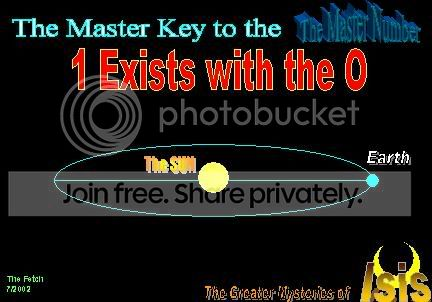The Pi Proportion and the Ordering of the Universe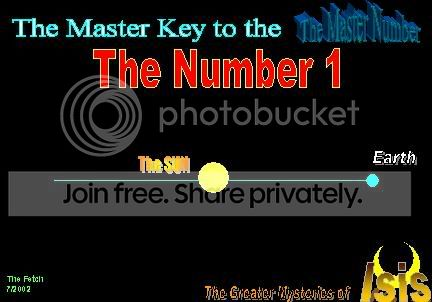When we look at the process of "constructing this really divine system", it is impossible to do so without the Pi proportion. As referenced above, there is an intrical link between observable "cycles" of the Universe as viewed from Earth.

The easiest way to comprehend this is to translate the concept of "cycles" into the word "orbits". Orbits can be divided into their respective Pi proportions through the establishment of key marking points in the observation process.

For example:

The period from a New Moon to a Full Moon effectively divides the orbit of the Moon as it circles the Earth in half relative to the time it takes for the Moon to complete one orbit around the Earth. The "1", or "diameter component" of the "Pi proportion" would then be the orbital time divided by 2.

The Synodic Orbit of the MOON is 27.3 days. Divide 27.3 days by 2 to arrive at the "Pi proportion", or the "diameter", which reveals a formula of 13.65. Check your numbers against the Isisian Codes to correctly encode, or construct, the word for MOON.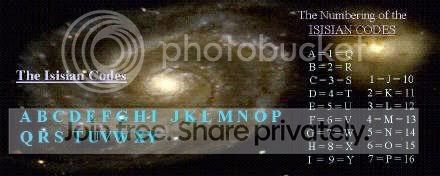The Letter M has a value of 13.
The Letter O has a value of 6
The Letter N has a value of 5.

The Formula for MON is M.ON = 13.65 days from New Moon to Full Moon. As the orbit of the MOON is not constant, but changes, the word MOON would simply be set at a formula of 13.665 days, allowing for orbital tolerance.

The Word SUN reveals the formula of

S = 3
U = Sum of 1:6=21 Letter U
N = 5

or, more simply SUN = 365

EARTH = 5+1+18+20+8 = 52 Weeks, or the "circumference" of the "Pi proportion".

The Period from Winter Solstice to Summer Solstice is 52 weeks divided by 2 (in half) to reveal 52/2 = 26 weeks. This is the number of Letters to the Alphabet, which should be a clue enough, however, the Isisian Code words for this period is

GOD = 7+15+4 = 26 weeks

Since "GOD" is "masculine", and GODDESS is "feminine", then GODDESS would, by necessity, have a value that equates to the Pi Proportion. This Pi proportion would have to be a circumference reading, and so the cycle time, being observable and hence known, would then be encoded into the word. The proper way (through the use of Art) to decode this (or encode this, as it were), is to understand that the 1 and the 0 exist together. This is a conceptual reality, so words that are feminine will often include the masculine as designed (another observable conceptual reality): SHE contains HE, HER contains HE, WOMAN contains MAN.

There is an esoteric coding in play.

The male is the diameter code while the female component of the word incorporates the circumference of the Pi proportion.

In the word GODDESS, GOD is the DIAMETER while DESS is the CIRCUMFERENCE.

DESS reveals the following digits:

D = 4
E = 5
S = 19
S = 19

The formula, then, is easy to ascertain.

(S*S)+D = (19*19)+4 = 365 days; or
(S*S)+E = (19*19)+5 = 366 days

What is evolving is that the Universe is being set into a form of ORDER, and the order is appearing from the seeming CHAOS of the ALPHABET

The Great Year by Plutarch is said to be 25920 years. The PI proportion, comprised as it from a "diameter" and "circumference" component, would have as its circumference component the period of 25920 years, while the diameter component would have as its value a period of 25920/2 = 12960 years.

The Pi Proportion for the Great Year, or 25920 years is the word HELL. That is right. HELL.

H = 8 = 36
E = 5
L = 12
L = 12

HELL = (H*E*L*L) = 36*5*12*12 = 25920 Years

Of course, the idea of equating Letters to Numbers should not be a secret to anyone who is familiar with the United States Monetary System. The coding is there right before your very eyes.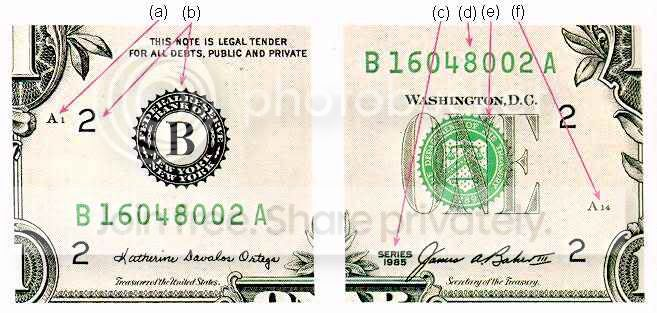Notice that the "B" is the 2nd letter of the alphabet and there is a 2 located as referenced. Study any US currencly, and this pattern will be found. Where you find an L you will also see the number 12, as 12 is the 12 Letter of the Alphabet. A "G" will have a "7", and an "I" a "9" and so forth.

So when you take the diameter (Pi proportion) of the Great Year of 25920/2 = 12960, this creates the Isisian Code of

L = 12
I = 9
F = 6

and E, having a right to remain silent as E is 5 and 1+2+3+4+5 = 15 = O Letter, the E has a value of O to show the proper Pi proportion formula.

LIFE = 12960 Years = 1/2 of the time of the Great Year.

I hope you have found this to be an entertaining and interesting read into the Ordering of the Universe using Esoteric English and higher level Gematria.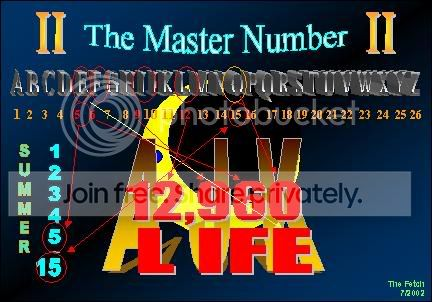Anonymous said...

what a beautiful article.

this one is the one that just knocked me off my rocker. lol

it put everything into place - for me.

that is why when i did a SAY TEN
it was a EUREKA moment for me!

it was the HELL part i was missing

wow!

may Gods bless you!
thank Q

!!!!!Anonymous said...

Are you aware of these codes having been used in the book of Revelation? Also,as the messenger,does your name mean anything?Anonymous said...

if i bonk myself again ill get a headache !

25920 is the circumference, the female, the year. just like 365 is.

it is 25920 as BEIT, YIT (white)

"the white house", "the great white lady"

"it aint over till the fat lady sings"

cute

-sky-

Indras Net said...

Great work man, this is my kind of work here. Are you aware of the Mathmatic formula expressed by Hebrew Gematria in Genesis that in 3D represents the Toroid or the shape of the Universe. And that the least denominable representation of the form of the toroid when held in front of a flashlight forms shadows of every single letter of the Hebrew Alphabet. Check it out Here Keep up the great work man, I look forward to more

MAYA said...

This is very interesting article,I loved your reserch.
I started wonder so how can I apply to our alphabet,well,it's not mathematical like alphabets but more visionary.
Anyway the symbols are made by ancient's knowledge from the essence of Universe,this is true.Weather mathematical or visionary,they are hidding the essences of our surroundings,and ourselves.....Have a nice day;-)

Gary Val Tenuta said...

This Isisian code is interesting. Not sure I fully understand the system but I'll give it a closer look.

I've been exploring the English gematria for over a decade and have developed a website based on the work. Of particular interest (to those of you who are interested in the encoded English alphabet) is a table I developed. I call it the AlphaNumber Table. It came together when I converted the numbers 0 through 9 into the words ZERO through NINE and calculated the alphanumeric values of each of those words. The result of this work was quite stunning. You can see the AlphaNumber Table and the complete explanation of it here:

http://www.secretofnine.com/alphatable.html

Regarding the info here (on the Illuminatus Observer page) about the Pi-proportion, it's interesting to note the discussion on the 2nd page of the AlphaNumber Table where I show how the Table relates to 360, the number of arc degrees in a complete circle.

thewillwillwill said...

Please clarify why the U in SUN is a 6, why the H in HELL is 36?Anonymous said...

Hi from -sky-

@thewillwillwill

IF I may offer some information?

The letter "U" is in the 21 spot of the English Alphabet correct?

The letter F is located at the number 6 spot correct?

So, when he is talking about "summative values" he is referring to the sum of the number/letter added up for - whichever particular number/letter he is referring to.

For example:

The letter B.
Holds its position as the second letter of the English Alphabet. Therefor is holds the number 2 spot.

What is the summative value of the number 2?

It is 3.
How?

2 is representing its summative value as 3 by following the summative operations. Adding up the numbers before 2 and to include 2.

1+2 is the summative value FORMULA for arriving at 3.

1:2 represents this concept.

1:2=3
1+2=3

Now if you use the number 6 you will find:

1+2+3+4+5 are all the numbers that come before 6 correct? Now include 6.

1+2+3+4+5+6 What is that total summative value?

Did you get 21?

So if you were to say -- "The letter U as 21 represents F as 6 you are pointing out that the letter U is equal to the summative value of 6.

The letter U also represents 231 as 1:21=231. 1+2+3+4+5+6+7+8+9+10+11+12+13+14+15+16+17+18+19+20+21=231

It is like saying 2/4 = 1/2

They are the same thing only one is reduced.

As for the letter H being 36 - You need to find the value for the letter H.

Which is 8.
1:8=36
1+2+3+4+5+6+7+8=36

Once that is done you can see how the word HELL = 25920

H=36
E=5
L=12
L=12

36 x 5 x 12 x 12 = 25920

36*5=180 (Half of 360)
180*12=2160 (the years of each Zodiac)
2160*12=25920 (The years of each Zodiac multiplied by how many Zodiac signs there are and that equals "The Great Year")

IMHO We could always place the E as a 0 and use that formula as 360*12*12 but,

the E as 5 is not silent (1+2+3+4+5=15=0) and is sounded out in the word HELL so we have to arrive with a formula that is solid to show and express this.

I hope that helps.

-sky-Anonymous said...

@sky Thanks. I get the reverse summative thing now.. It had occurred to me that old books and prints the U and V are interchangeable, so a 5 can be a 6 and a 6 can be a 5... The sum of 1-5 = 15 1+5=6..

WORD 5 6 9 4,, 5x6x9x4=1080(radius of moon, 1080 parts per hour of Hebrew Cheilek)
5+6+9+4=24(hour in day, day is as 1000 years) 24 x 1080 = 25920Precession. If GOD is the Word which is 25920 and Hell is 25920. In effect the cycle is both heaven and hell. It is the duality.

Simple(6,74) English(7,74) Gematria(8,74): GOD=7_4

'The key'(74): A=1, B=2...Z=26. The only irregularity is O: the circle can be the 15th letter or zerO or _.

GOD=7_4=11/26
religion=74/89=R18+E5+L12+I9+G7+I9+O(15)+N14
Osiris=74/89=O(15)+S19+I9+R18+I9+S19

The GOD=7_4 algorithm was used to create this universe and the Plan-it Earth...

7 continents & 4 seasons, ('7 Seas') & 4 oceans. ~74% of Earth's surface is water. '7 Classical Planets': 4 don't cast shadows on Earth (Venus does)/4 can't be seen during day (Venus can be). 4 lunar phases of roughly 7 days (~7.4) each. Lunar year + 7 day week + 4 days = 365 day solar year. The Big Dipper is 7 stars with 4 forming rectangular ladle. The 4th star in the middle is the brightest. The Big Dipper points towards the North Star which is the tip of the handle of the Little Dipper which is also 7 stars with 4 stars making up its ladle. Mars has a 47-year cycle. Etc.

See my tweaked NASA conference presentation of 4/21/09 at http://PlanetNestor.blogspot.com .

Unknown said...

They should make this a bit smaller so as to be unreadable.

But how many adults have missed that thing with the fiat notes!?

At first glace it seems like quite the significant find. It seems to lend credence however to the import of the letters... But recall how They seem to have a fetish in placing their symbols everywhere.

We see now what is goes back to. I've still to hear mention of Application.

Without this application, it seems to me there is a possibility that everything discussed here, as well as all those other symbols and memes etc., allude to a Contrived reality.

It's like they Must throw this stuff out everywhere; as a magical act to keep Their reality in place.

Also note the comments above. Yet another guy has found patterns using his own system. Yet Another system finding stuff.

I'm familiar with both Stan and Dan's work with Hebrew letters. Now That body of research seems to indicate the a certain form of Hebrew trumps all the above!

Billy said...

Sir, do learn more about Pi please look into the lecture video from super teacher Les Brown on YouTube called,” The Physics of Crystals “. Les authored a book available on Scribd called “ The Pyramid “.

Every shape is found within ANY shape.